Symbol
ProblemWhat's New Can You Show Me the $Nay7$ Complete the table of values of the exponential function. Then, draw its graph $in$ the given coordinate plane. $x$ $-3$ $-2$ $-1$ $0$ $1$ $2$ $3$ $f\left(x\right)=3^{x}$
10th-13th grade
Other
Search count: 109
SolutionQanda teacher - TeacherP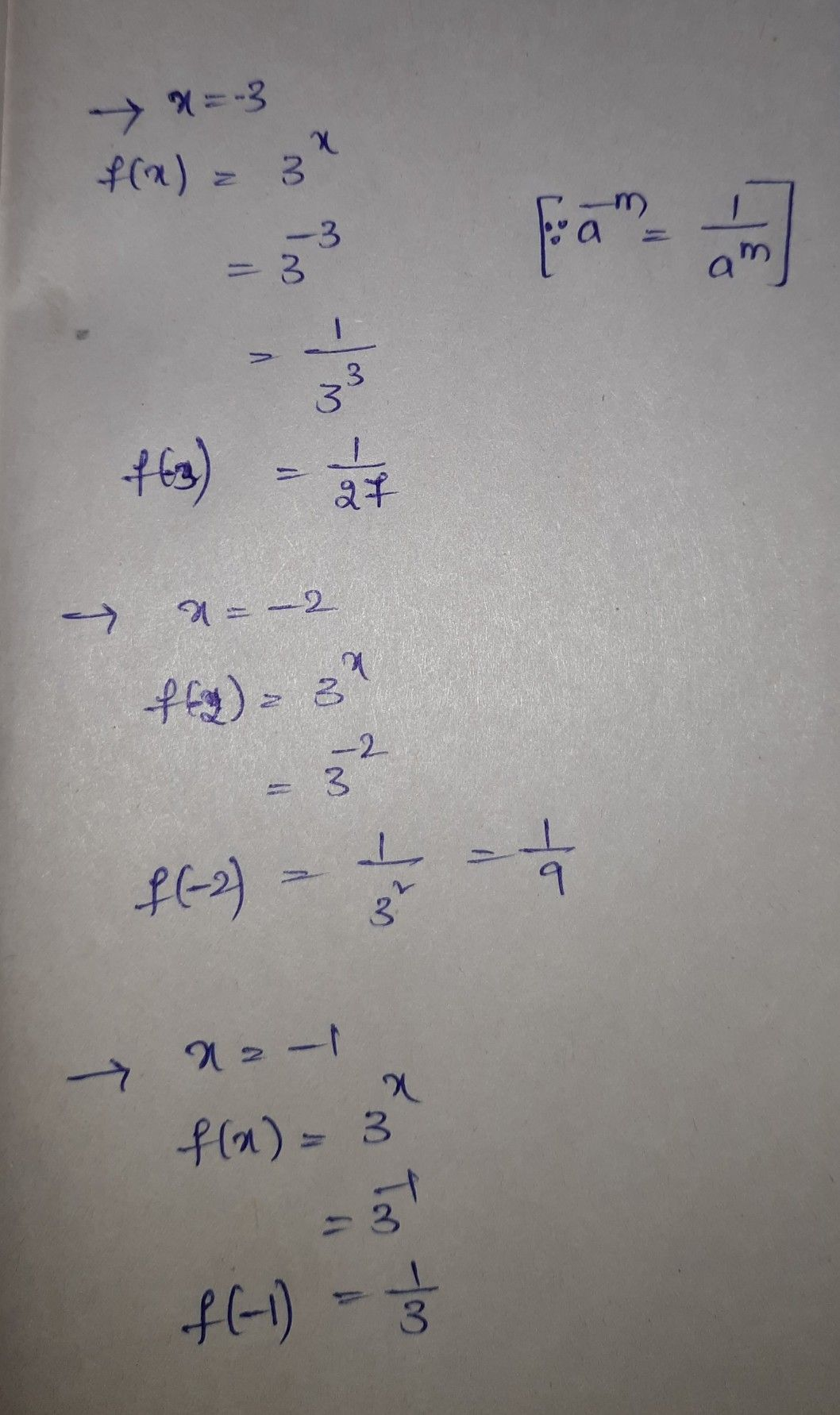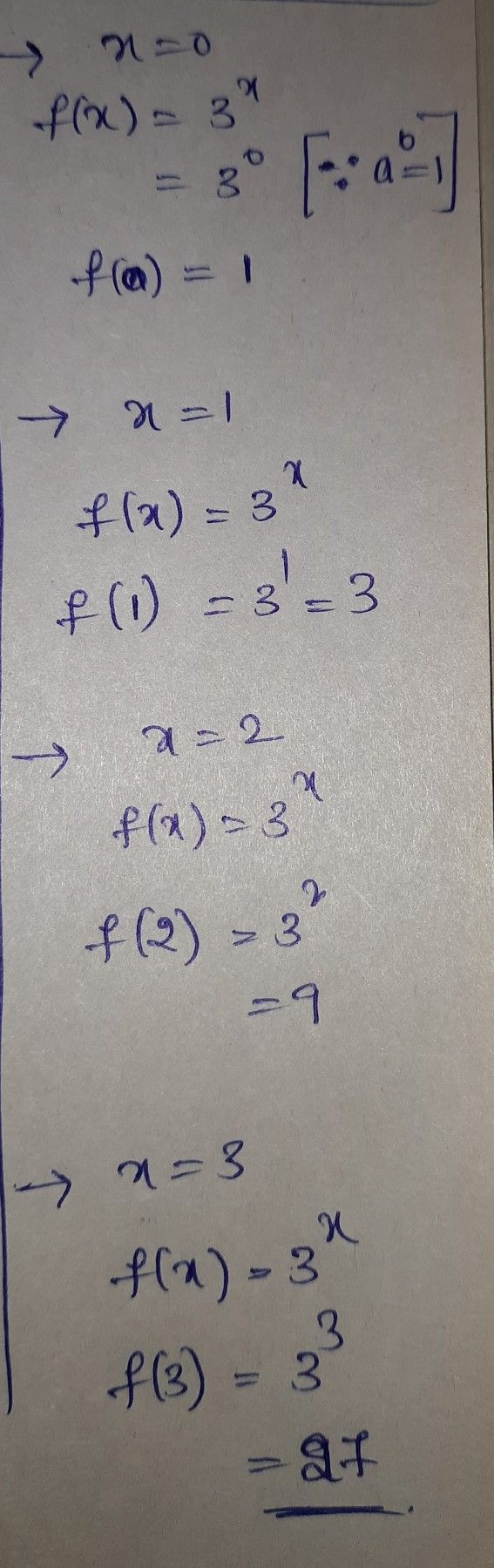Student
how to arrange?Qanda teacher - TeacherP
waitStudent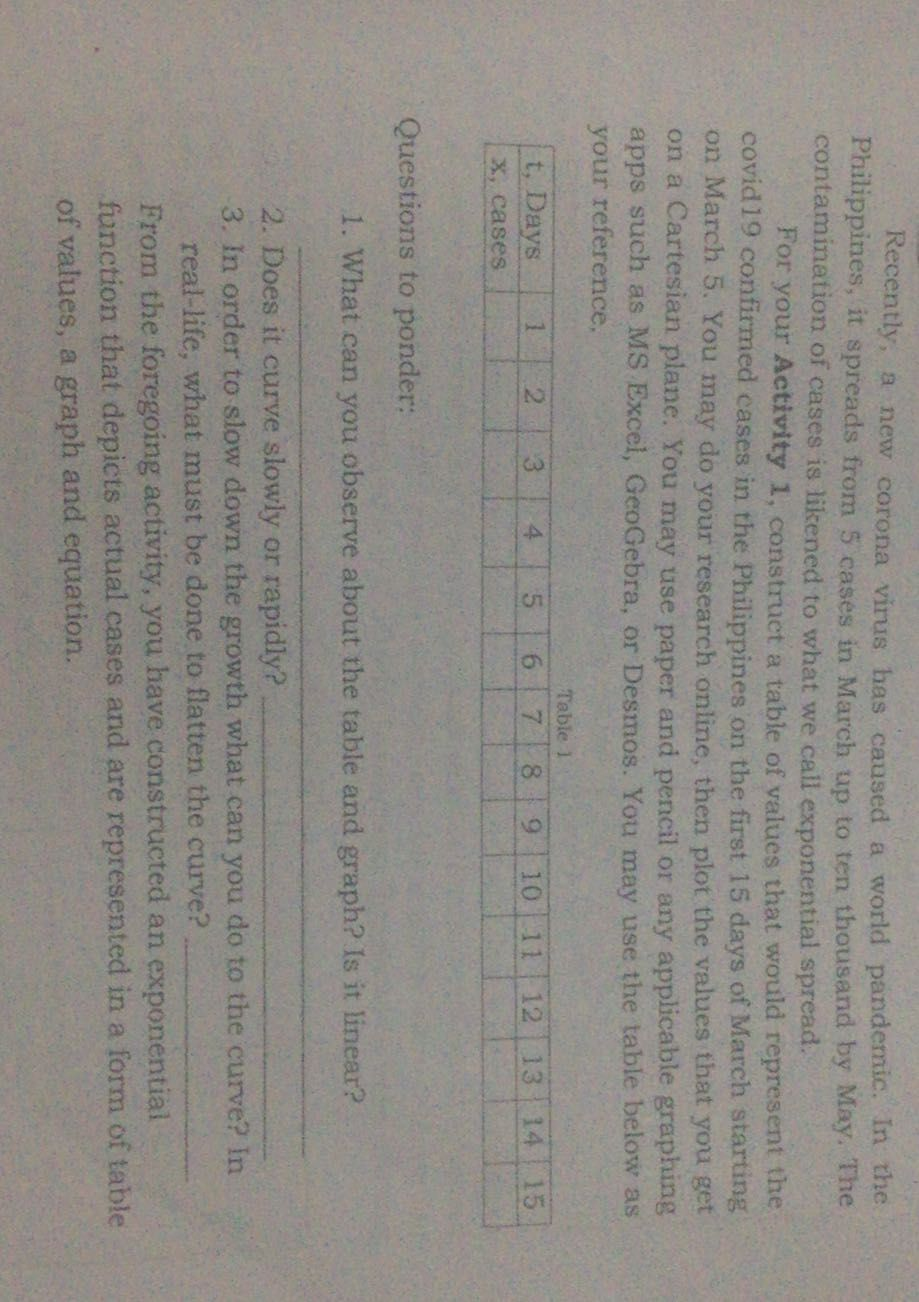okey i waitQanda teacher - TeacherP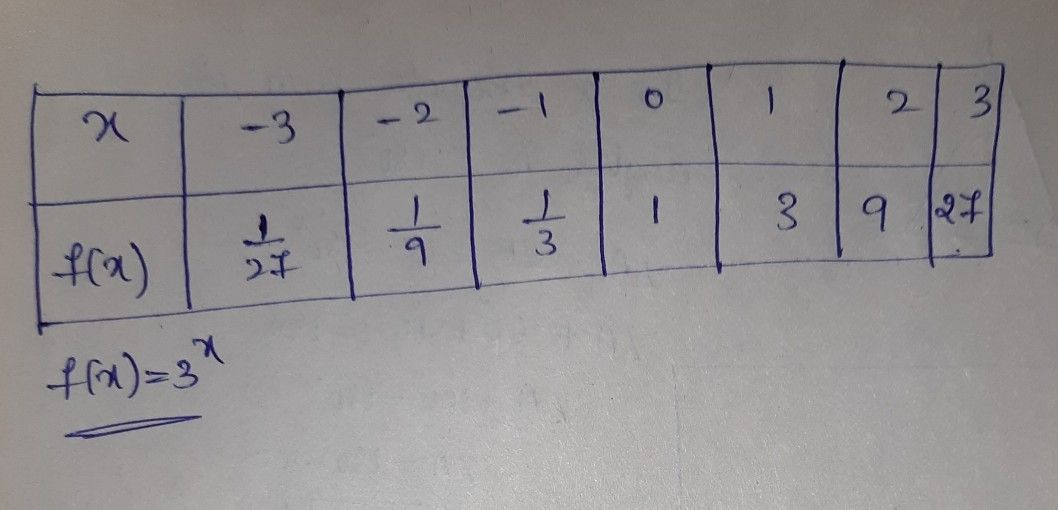Student
Question answe please
her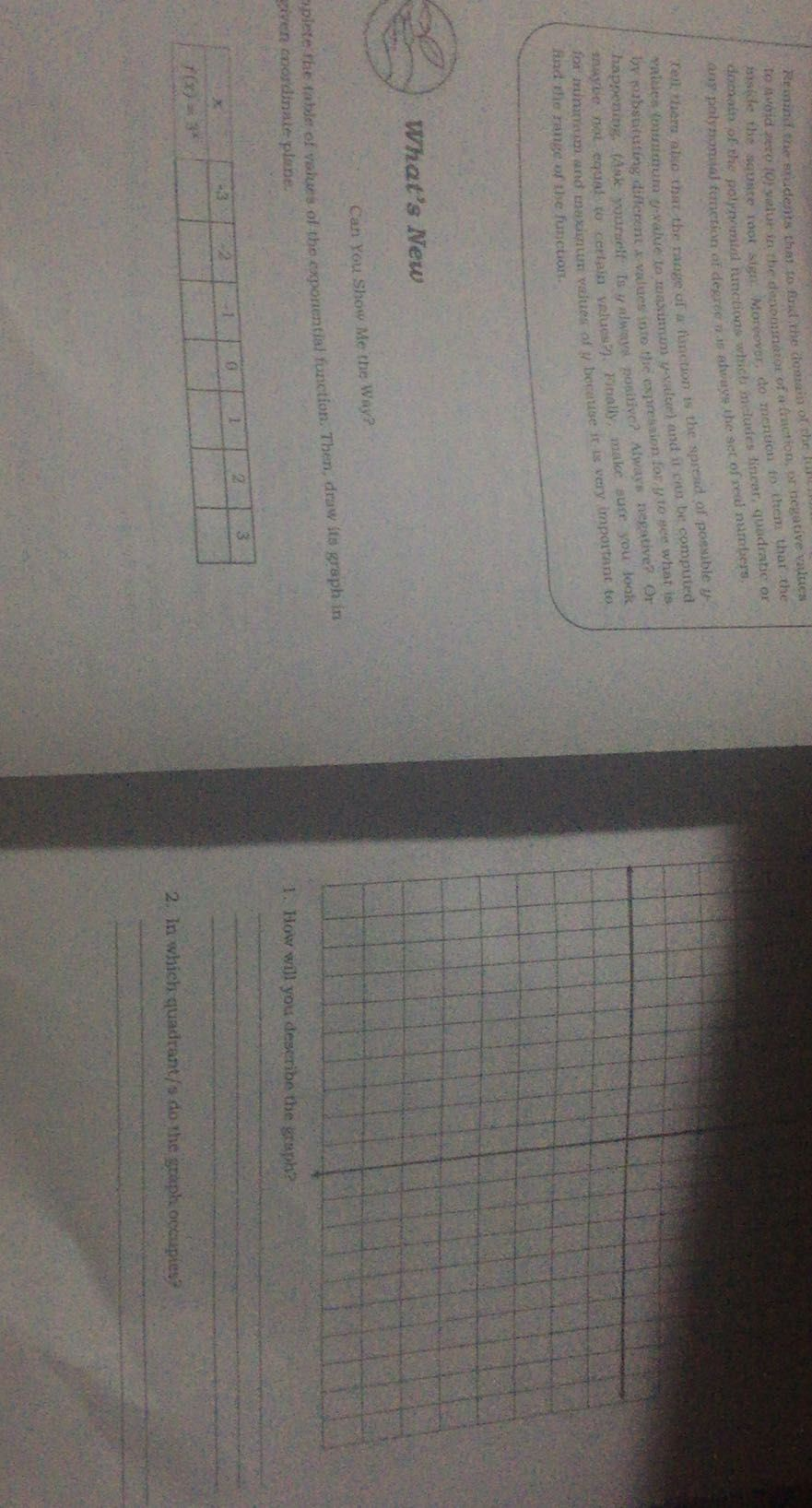Qanda teacher - TeacherP
for your first question, this is the final arrangementStudent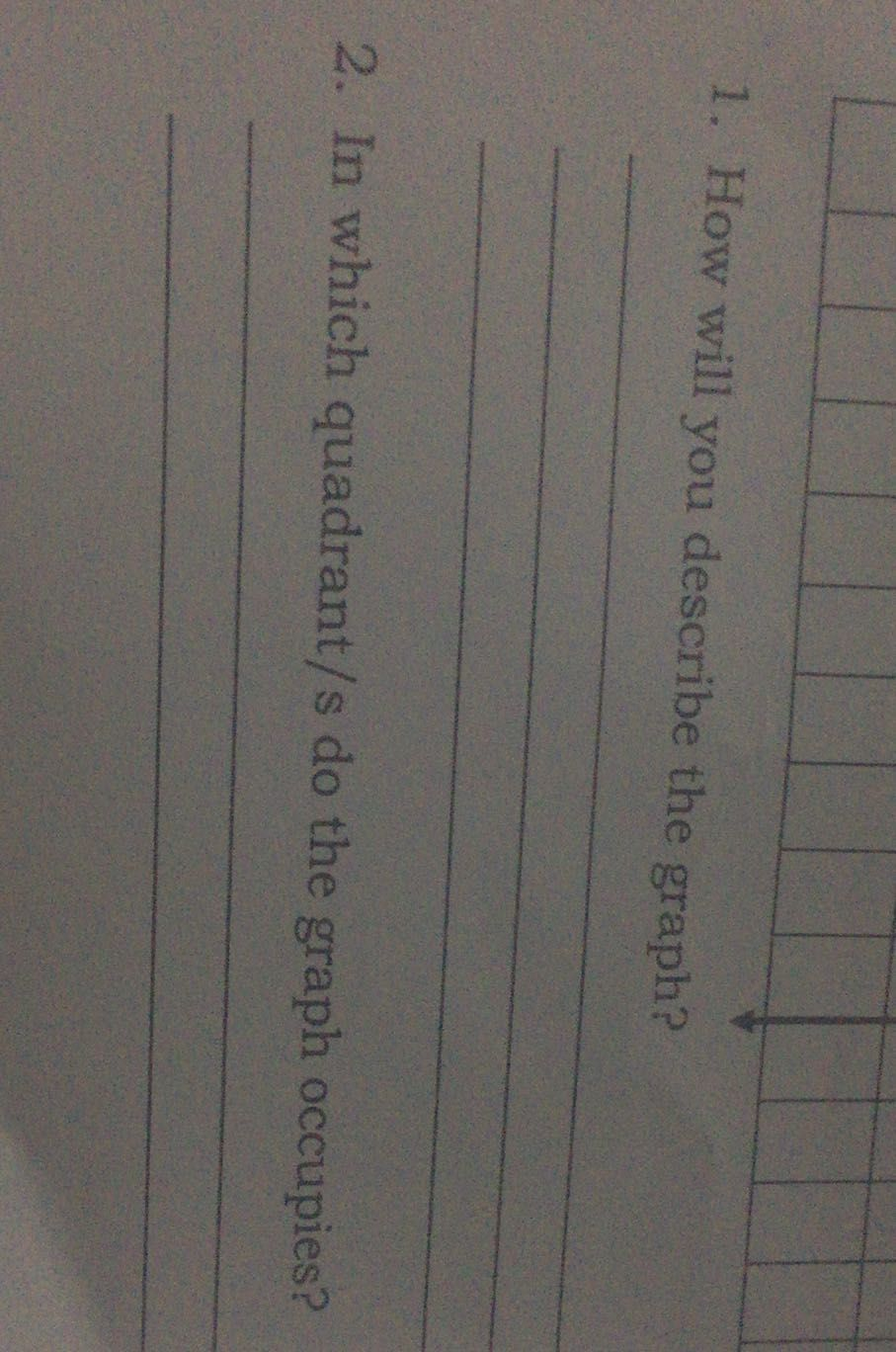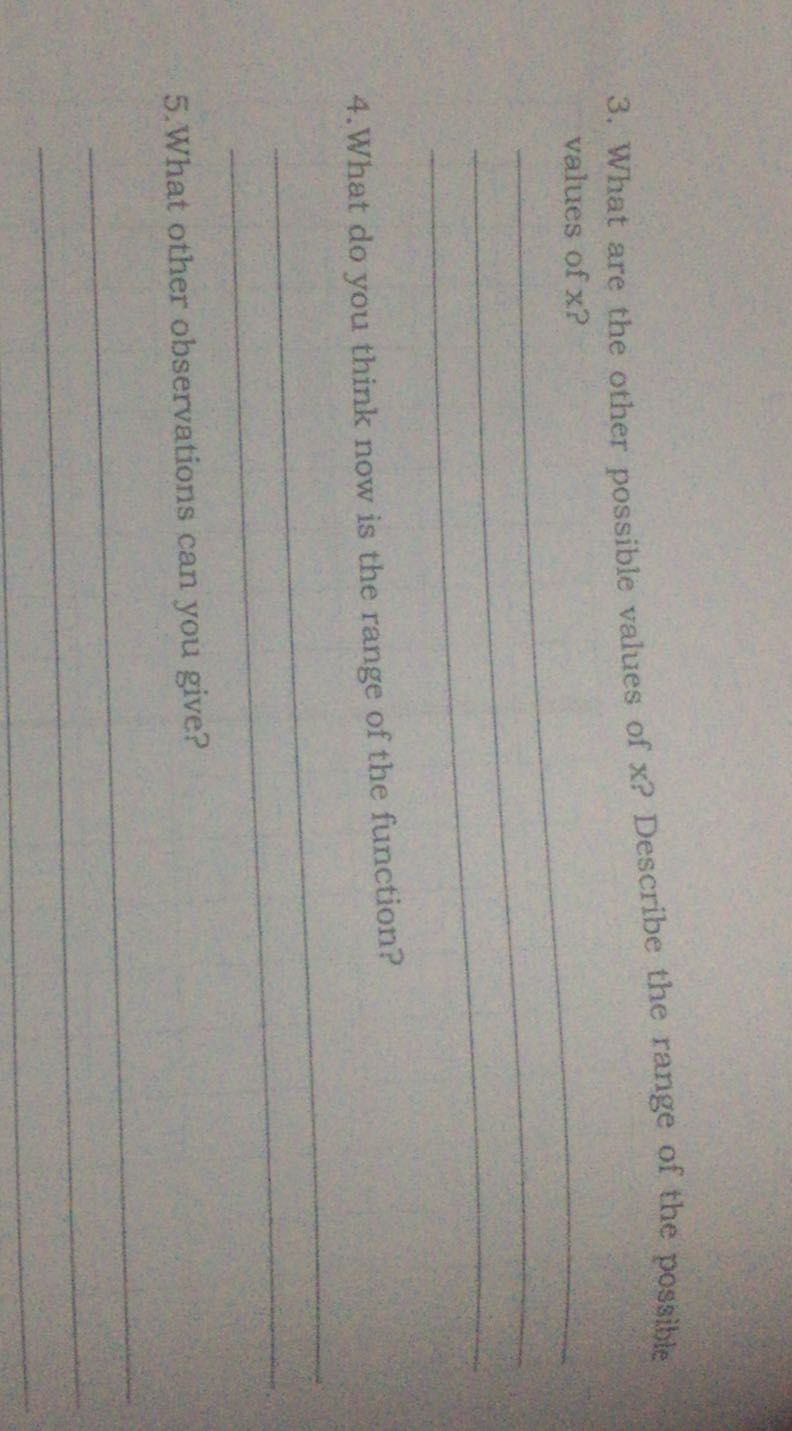continuation of my answer to you